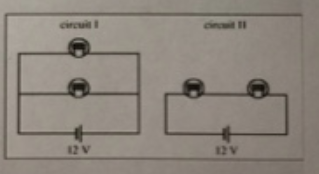# Problem: The lightbulbs in the circuits below are identical with the same resistance R. Which circuit produces more light? (brightness ⇐ ⇒ power)a) circuit Ib) circuit IIc) both the samed) It depends on R

###### FREE Expert Solution

In a parallel connection, as shown in circuit I, the voltage is the same for both bulbs.

The light bulbs have the same resistance.

The power of each bulb will be given by:

Pp = V2/R

However, suppose the bulbs are connected in series like in the second case, the current through each bulb will be the same as the current through the entire circuit.

This current is given by:

$\mathbit{i}\mathbf{=}{\mathbit{i}}_{\mathbf{1}}\mathbf{=}{\mathbit{i}}_{\mathbf{2}}\mathbf{=}\frac{\mathbf{V}}{{\mathbf{R}}_{\mathbf{e}\mathbf{q}}}$

90% (353 ratings)###### Problem Details

The lightbulbs in the circuits below are identical with the same resistance R. Which circuit produces more light? (brightness ⇐ ⇒ power)a) circuit I

b) circuit II

c) both the same

d) It depends on R